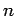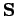Next: General Principles Up: Addition of Angular Momentum Previous: Addition of Angular Momentum

# Introduction

Consider an electron in a hydrogen atom. As we have already seen, the electron's motion through space is parameterized by the three quantum numbers,, and(see Sect. 9.4). To these we must now add the two quantum numbersandwhich parameterize the electron's internal motion (see the previous chapter). Now, the quantum numbersandspecify the electron's orbital angular momentum vector,, (as much as it can be specified) whereas the quantum numbersandspecify its spin angular momentum vector,. But, if the electron possesses both orbital and spin angular momentum then what is its total angular momentum?

Richard Fitzpatrick 2010-07-20